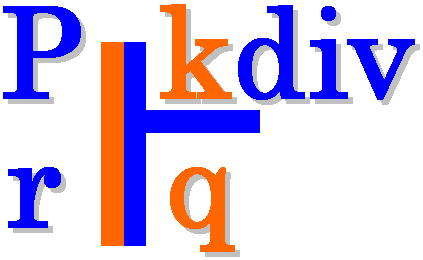# kdiv user manual# 1. Introduction

kdiv is a generator for routines for optimized division by an integer constant. It can be used for calculating an integer division with the routines presented in Henry S. Warren's "Hacker's Delight" book. kdiv can also be used for emitting a NAC (generic assembly language) or ANSI C implementation of the division.

# 2. File listing

The kdiv distribution includes the following files:

# 3. Installation

There exists a quite portable Makefile (Makefile in the current directory). Running make from the command prompt should compile kdiv.

# 4. Prerequisites

• [mandatory for building] Standard UNIX-based tools
• gcc (tested with gcc-3.4.4 on cygwin/x86)
• make
• bash

# 5. kdiv usage

The kdiv program can be invoked with several options (see complete option listing below). The usual tasks that can be accomplished with kdiv are:

• test signed/unsigned division by constant
• generate a NAC optimized software routine for the division
• generate an ANSI C optimized software routine for the division.

ANSI C routines have been tested only for a width of 32-bits (see option below).

kdiv can be invoked as:

\$./kdiv [options]

The complete kdiv options listing:

-h
Print this help.
-d
Enable debug/diagnostic output.
-errors
Report only inconsistencies to the expected division results.
-div <num>
Set the value of the divisor (an integer except zero). Default: 1.
-width <num>
Set the bitwidth of all operands: dividend, divisor and quotient. Default: 32.
-lo <num>
Set the lower integer bound for dividend testing. Debug output (-d) must be enabled. Default: 0.
-hi <num>
Set the higher integer bound for dividend testing. Debug output (-d) must be enabled. Default: 65535.
-signed
Construct optimized routine for signed division.
-unsigned
Construct optimized routine for unsigned division (default).
-nac
Emit software routine in the NAC general assembly language (default).
-ansic
Emit software routine in ANSI C (only for width=32).

Here follow some simple usage examples of kdiv.

1. Generate the ANSI C implementation of n/11 optimized routine.
\$ ./kdiv -div 11 -width 32 -unsigned -ansic
1. Generate the NAC implementation of n/(-7) optimized routine.
\$ ./kdiv -div -7 -width 32 -signed -ansic

3. Generate the ANSI C implementation of n/23 optimized routine. Also run some tests with an internal generator for the dividend range [0..1024].

\$ ./kdiv -div 23 -width 32 -unsigned -ansic -d -lo 0 -hi 1024

# 6. Quick tutorial

kdiv can be used for arithmetic optimizations in user programs. Assume the following user program (test.c):

```// test.c
#include <stdio.h>
#include <stdlib.h>
int main(int argc, char *argv[]) {
int a, b;
a = atoi(argv);
b = a / 23;
printf("b = %d\n", b);
return b;
}
```

This file is compiled and run as follows with one additional argument:

\$ gcc -Wall -O2 -o test.exe test.c
\$ ./test.exe 155

and the expected result is:

\$ b = 6

The user can apply kdiv for generating a constant division routine for a/23:

\$ ./kdiv -div 23 -width 32 -signed -ansic

and the corresponding routine is produced. Then, the user should edit a new file, let's say test.opt.c and include the produced routine. The resulting optimized source file should be as follows:

```// test.opt.c
#include <stdio.h>
#include <stdlib.h>
inline signed int kdiv_s32_p_23 (signed int n)
{
signed int q, M=-1307163959, c;
signed long long int t, u, v;
t = (signed long long int)M * (signed long long int)n;
q = t >> 32;
q = q + n;
q = q >> 4;
c = n >> 31;
q = q + c;
return (q);
}

int main(int argc, char *argv[]) {
int a, b;
a = atoi(argv);
b = kdiv_s32_p_23(a);
printf("b = %d\n", b);
return b;
}
```

This file is compiled and run as follows with one additional argument:

\$ gcc -Wall -O2 -o test.opt.exe test.opt.c
\$ ./test.opt.exe 155

The target platform compiler (e.g. gcc or llvm) is expected to inline the kdiv_s32_p_23 function at its call site.

# 7. Running tests

In order to build and run a series of sample tests do the following:

\$ ./build.sh
\$ ./test.sh

# 8. Contact

You may contact me for further questions/suggestions/corrections at: